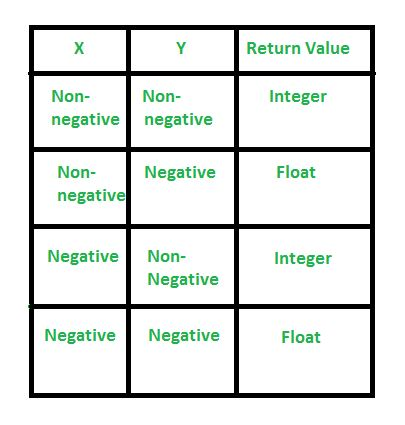Related Articles

# pow() in Python

• Difficulty Level : Basic
• Last Updated : 21 Jan, 2021

Python offers to compute the power of a number and hence can make the task of calculating the power of a number easier. It has many-fold applications in day to day programming.

## Python3

 `# Python code to demonstrate naive method``# to compute power``n ``=` `1``for` `i ``in` `range``(``1``,``5``):``    ``n``=``3``*``n` `print` `(``"The value of 3**4 is : "``,end``=``"")``print` `(n)`

Output:

`The value of 3**4 is : 81`

## Using Pow() Function

1. float pow(x,y): This function computes x**y. This function first converts its arguments into float and then computes the power.

Syntax: float pow(x,y)

Parameters :

x : Number whose power has to be calculated.

y : Value raised to compute power.

Return Value :

Returns the value x**y in float.

## Python3

 `# Python code to demonstrate pow()``# version 1` `print` `(``"The value of 3**4 is : "``,end``=``"")` `# Returns 81``print` `(``pow``(``3``,``4``))`

Output:

`The value of 3**4 is : 81.0`

2. float pow(x,y,mod) : This function computes (x**y) % mod. This function first converts its arguments into float and then computes the power.

Syntax: float pow(x,y,mod)

Parameters :

x : Number whose power has to be calculated.

y : Value raised to compute power.

mod :  Value with which modulus has to be computed.

Return Value :

Returns the value (x**y) % mod in float.

## Python3

 `# Python code to demonstrate pow()``# version 2` `print` `(``"The value of (3**4) % 10 is : "``,end``=``"")` `# Returns 81%10``# Returns 1``print` `(``pow``(``3``,``4``,``10``))`

Output:

`The value of (3**4) % 10 is : 1`

#### Implementation Cases in pow() :## Python3

 `# Python code to discuss negative``# and non-negative cases` `# positive x, positive y (x**y)``print``(``"Positive x and positive y : "``,end``=``"")``print``(``pow``(``4``, ``3``))` `print``(``"Negative x and positive y : "``,end``=``"")``# negative x, positive y (-x**y)``print``(``pow``(``-``4``, ``3``))` `print``(``"Positive x and negative y : "``,end``=``"")``# positive x, negative y (x**-y)``print``(``pow``(``4``, ``-``3``))` `print``(``"Negative x and negative y : "``,end``=``"")``# negative x, negative y (-x**-y)``print``(``pow``(``-``4``, ``-``3``))`

Output:

```Positive x and positive y : 64
Negative x and positive y : -64
Positive x and negative y : 0.015625
Negative x and negative y : -0.015625```

This article is contributed by Manjeet Singh. If you like GeeksforGeeks and would like to contribute, you can also write an article using contribute.geeksforgeeks.org or mail your article to contribute@geeksforgeeks.org. See your article appearing on the GeeksforGeeks main page and help other Geeks.